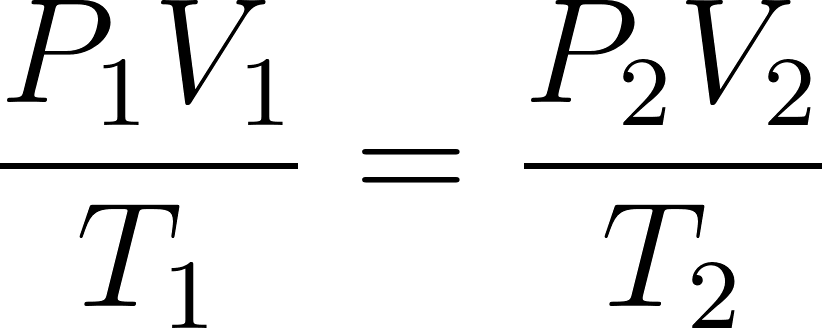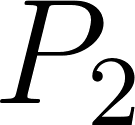×
Get Full Access to Introductory Chemistry - 5 Edition - Chapter 11 - Problem 51p
Get Full Access to Introductory Chemistry - 5 Edition - Chapter 11 - Problem 51p

×

# Solved: A sample of gas with an initial volume of 28.4 L at a pressure of 725 mm Hg andISBN: 9780321910295 34

## Solution for problem 51P Chapter 11

Introductory Chemistry | 5th Edition

• Textbook Solutions
• 2901 Step-by-step solutions solved by professors and subject experts
• Get 24/7 help from StudySoup virtual teaching assistantsIntroductory Chemistry | 5th Edition

4 5 1 328 Reviews
20
0
Problem 51P

PROBLEM 51P

A sample of gas with an initial volume of 28.4 L at a pressure of 725 mm Hg and a temperature of 305 K is compressed to a volume of 14.8 L and warmed to a temperature of 375 K. What is the final pressure of the gas?

Step-by-Step Solution:

Problem 51P

A sample of gas with an initial volume of 28.4 L at a pressure of 725 mm Hg and a temperature of 305 K is compressed to a volume of 14.8 L and warmed to a temperature of 375 K. What is the final pressure of the gas?

Step by step solution

Step 1 of 2

According to the combined gas law,.....................(1)

Rearrange the equation (1)............................(2)

Here,= Initial pressure of the gas= Initial volume of the gas= Initial temperature= final pressure of the gas= final volume of the gas= final temperature

Step 2 of 2

##### ISBN: 9780321910295

Unlock Textbook Solution